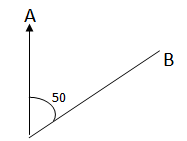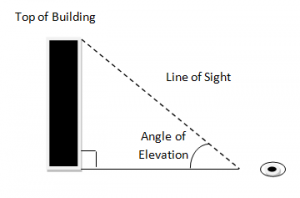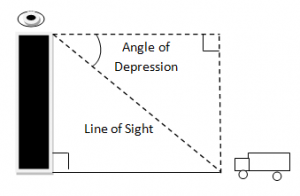Friday , May 24 2019

# BearingsThe bearing of point B from another point A is:

• An angle measured north to at A.
• In a clockwise direction.
• Written as three- figured number (i.e from 000 to 300 degrees).

The bearing of B from A is 050 degree.

### Angle of Elevation:When a person looks at something above his or her location e.g (The top of a building). The angle formed between the horizontal ground and the line of sight is called the angle of elevation.

### Angle Of Depression:When a person looks at something below his or her location, the angle between the imaginative horizontal and vertical and the line of sight is the angle of depression.

Angle of depression is always of equal value as angle of depression because they are alternative angles.

### About Admin## Matrices

In Mathematics, matrices are arrays of numbers arranged in rows and columns. Types of Matrices: …